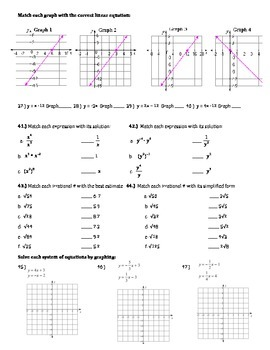# 8th Grade Math Common Core Review PacketSubject
Resource Type
File Type

PDF

(716 KB|4 pages)
Standards
Also included in:
1. Comprehensive review for preparing students for their 8th grade state assessment or end of year exam. - Game- Spiraled Review- Study Guides- Assessments- Differentiated Resources
\$37.45
\$19.99
Save \$17.46
• Product Description
• StandardsNEW
62 questions of 8th grade math common core review with answer key.
Topics covered:
- Simplifying and solving multi-step equations and inequalities
- Graphing linear equations and writing the equation of a line
- Transformations on the coordinate plane
- Systems of equations
- Pythagorean theorem
- Functions vs. non-functions
- Angle relationships
- Volume of a cone, cylinder, and sphere.
4 pages of comprehensive review. Great for a study guide, final exam, or diagnostic exam.

Check out my Exam Bundle for \$8.50. Includes an exam for each of the 7th grade math units, and a study guide that is aligned to each exam. Also includes a comprehensive final exam, and mid-term.
Understand that a function is a rule that assigns to each input exactly one output. The graph of a function is the set of ordered pairs consisting of an input and the corresponding output.
Know and apply the properties of integer exponents to generate equivalent numerical expressions. For example, 3² × (3⁻⁵) = (3⁻³) = 1/3³ = 1/27.
Know that numbers that are not rational are called irrational. Understand informally that every number has a decimal expansion; for rational numbers show that the decimal expansion repeats eventually, and convert a decimal expansion which repeats eventually into a rational number.
Verify experimentally the properties of rotations, reflections, and translations:
Total Pages
4 pages
Included
Teaching Duration
2 hours
Report this Resource to TpT
Reported resources will be reviewed by our team. Report this resource to let us know if this resource violates TpT’s content guidelines.